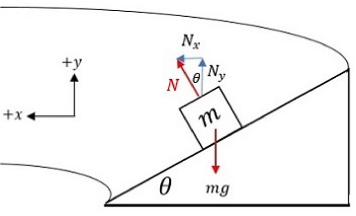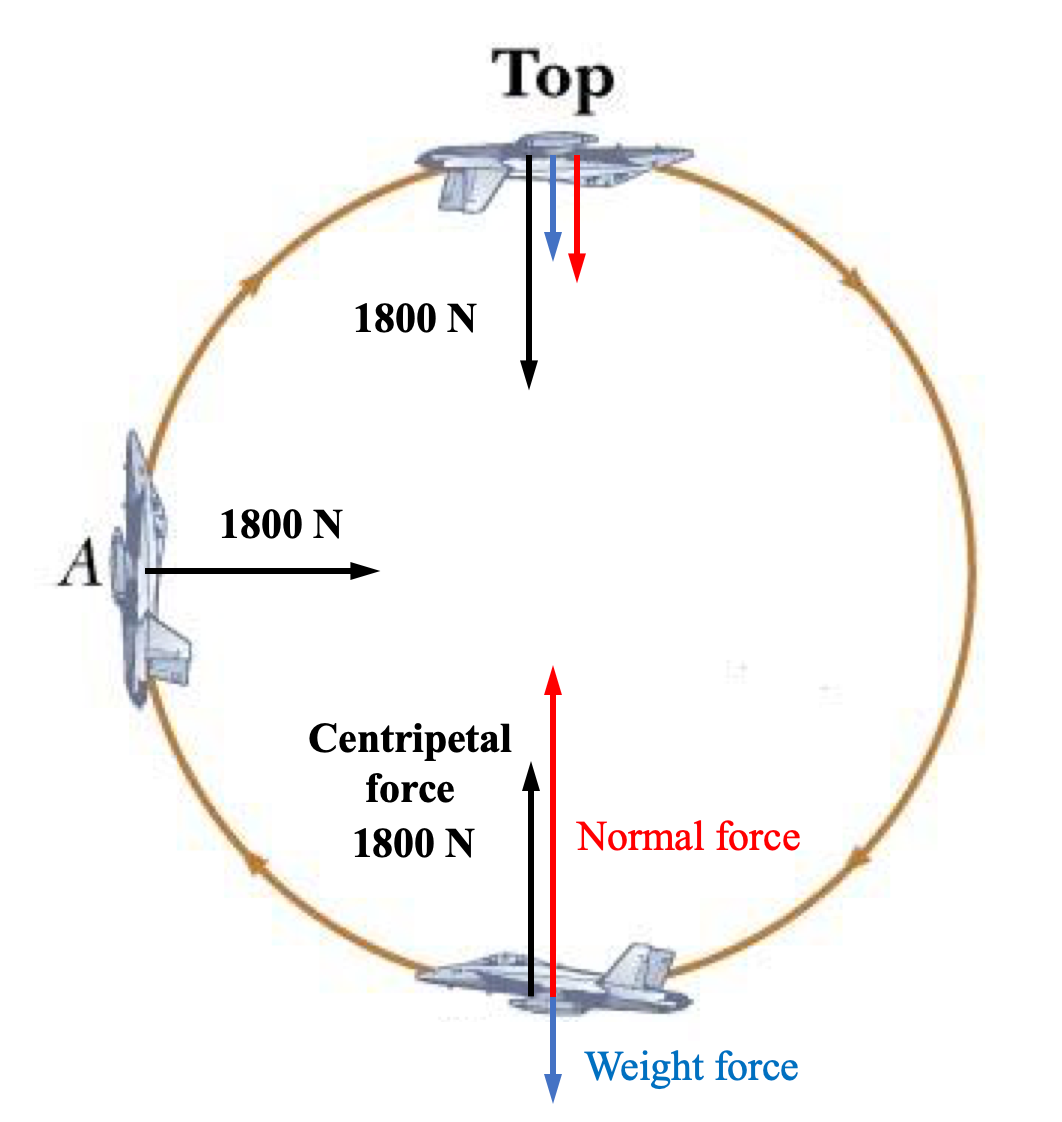# M5-S6: Circular Motion in a Variety of Situations

• ### analyse the forces acting on an object executing uniform circular motion in a variety of situations, for example:

- cars moving around horizontal circular bends
- a mass on a string
- objects on banked tracks

### Cars around horizontal circular bends

Centripetal force results from friction acting on the object towards ‘centre’ if the bend and orthogonal to the tangential velocity. Drivers or passengers in the car experience acceleration outwards because of inertia (not due to acceleration caused by centripetal force).

### Mass on a string

Centripetal force results from tension force acting on the object in the direction to where the rope is attached. This again, would be orthogonal to the direction of tangential velocity and towards centre of circular motion. However, in many cases since the mass is suspended during circular motion, the effect of gravity is made more obvious. Thus, weight force also acts on the mass which causes the mass to eventually decrease in precession

### Objects on banked surfaces

This scenario is similar to cars driving around a circular bend as identical forces are involved (friction, weight and normal force). However, in this case normal force is not equivalent to weight force so the object is more susceptible to inward force (motion). In addition to velocity, radius and mass, there are more factors which influence the object’s circular motion:

These include:

• angle of the banked surface to the horizon
• friction of the banked surfaceMathematical Proof:

Equating the horizontal component of normal force (N) to the centripetal force:

(1): Nsintheta=(mv^2)/r

Equating the vertical component of the normal force (N) to the weight force (mg)

(2): Ncostheta=mg

Now, by dividing equation (1) by (2), we obtain:

(Nsintheta)/(Ncostheta)=((mv^2)/r)/(mg)

This simplifies into:

tantheta=v^2/(rg)

rg*tantheta=v^2

Finally, we obtain the expression for tangential velocity required to complete uniform circular motion:

v=sqrt(rg*tantheta)

From this formula, we deduce that greater the angle of banked surface, greater the tangential velocity is required to undergo the circular motion. If the velocity is too small, the object succumbs to weight force by falling down the banked surface.

• The velocity required to complete circular motion on a banked surface is also lower than a horizontal bend of the same radius.

### Vertical circular motion

• In a gravitational field e.g. Earth, vertical circular motions are typically non-uniform (non-constant speed). This is because the centripetal force acting on the object changes throughout the vertical motion.
• However, vertical circular motions can be made uniform if the centripetal force (net force) acting on an object remains constant (but non-zero).For example, when an object attached to a rope is swung vertically, the tension in the rope changes throughout the motion. More tension force is needed at the bottom of the uniform circular motion compared to at the top because gravitational force acts in the opposite direction to centripetal force.

For uniform circular motion to occur, centripetal force must remain constant in magnitude.

 At the top of the circular motion: -(mv^2)/r=-mg-T_t   T_t=(mv^2)/r-mg At the bottom of the circular motion: (mv^2)/r=-mg+T_b   T_b=(mv^2)/r+mg

## Practice Question 1A fighter jet pilot of mass 70 kg completes a vertical loop-the-loop at a constant speed of 500 km/h, and with a radius of 750 m.

What normal force does he experience from his seat:

(a) at the top?

(b) at the bottom of the loop-the-loop?

## Solutions

First we can find the centripetal force by using the speed 500 km/hr. Remember speed must be in SI unit of m/s.

500   km hr^-1 = (500xx1000)/(60xx60)   ms^-2

v=139   ms^-2

F_c=(mv^2)/r

F_c=((70)*(139)^2)/750

F_c=1800  NAt the top of the loop-the-loop, the centripetal force is the sum of the pilot's weight force and normal force from the seat:

N_t=F_c-mg

N_t=1800-70xx9.8

N_t=1100  N downwards

At the bottom, the normal force is in the direction of the centripetal force again but weight force is now pointed away from the centre:

N_b=F_c+mg

N_b=1800+70xx9.8

N_b=2400  N  upwards

Therefore, it is evident that the normal force exerted by the seat on the pilot is much greater when the jet is at the bottom of the vertical loop. This is so that the net force (centripetal force) equals to that at the top of the loop and remains constant.

﻿Previous section: ﻿Introduction to Circular Motion

Next section: Quantitative Analysis of Circular Motion